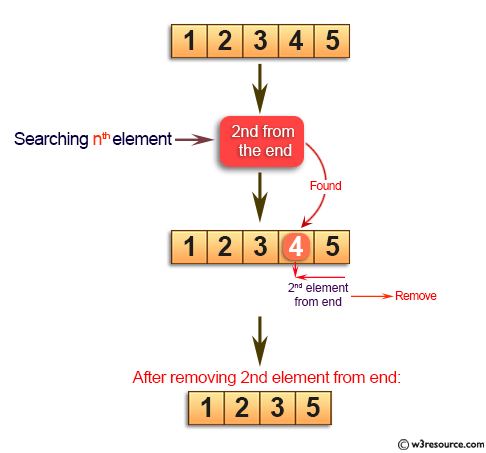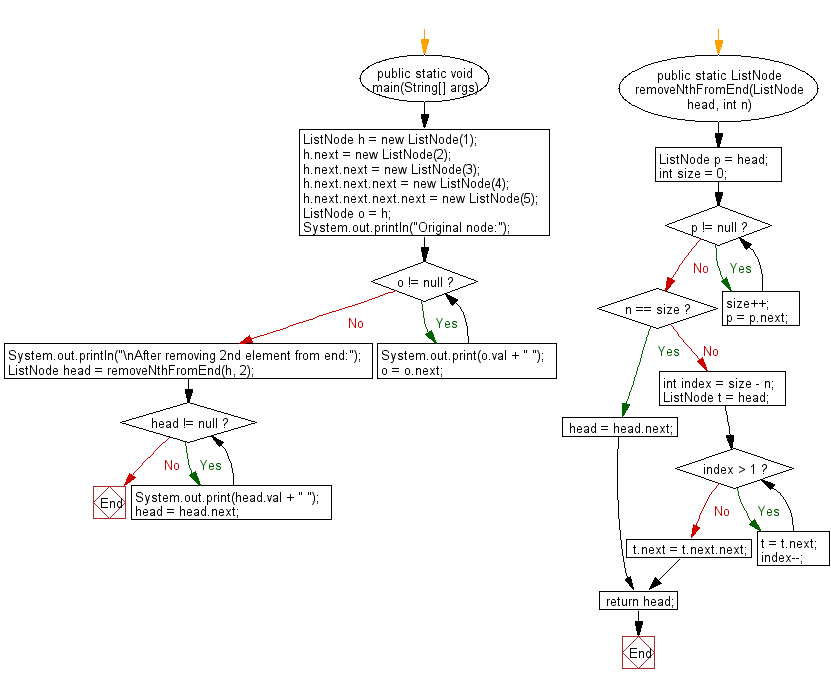﻿ Java: Remove the nth element from the end of a given list# Java Exercises: Remove the nth element from the end of a given list

## Java Basic: Exercise-145 with Solution

Write a Java program to remove the nth element from the end of a given list.

Pictorial Presentation:Sample Solution:

Java Code:

``````import java.util.*;
public class Solution {
public static void main(String[] args) {
ListNode h = new ListNode(1);
h.next = new ListNode(2);
h.next.next = new ListNode(3);
h.next.next.next = new ListNode(4);
h.next.next.next.next = new ListNode(5);
ListNode o = h;
System.out.println("Original node:");
while (o != null) {
System.out.print(o.val + " ");
o = o.next;
}
System.out.println("\nAfter removing 2nd element from end:");

}

}
public static ListNode removeNthFromEnd(ListNode head, int n) {
int size = 0;
while (p != null) {
size++;
p = p.next;
}
if (n == size) {
} else {
int index = size - n;
while (index > 1) {
t = t.next;
index--;
}
t.next = t.next.next;
}
}
}
class ListNode {
int val;
ListNode next;

ListNode(int val) {
this.val = val;
this.next = null;
}
}
```
```

Sample Output:

```Original node:
1 2 3 4 5
After removing 2nd element from end:
1 2 3 5
```

Flowchart:Java Code Editor:

What is the difficulty level of this exercise?

Test your Programming skills with w3resource's quiz.

﻿

## Java: Tips of the Day

getEnumMap

Converts to enum to Map where key is the name and value is Enum itself.

```public static <E extends Enum<E>> Map<String, E> getEnumMap(final Class<E> enumClass) {
return Arrays.stream(enumClass.getEnumConstants())
.collect(Collectors.toMap(Enum::name, Function.identity()));
}
```

Ref: https://bit.ly/3xXcFZt